python利用matplotlib进行可视化黎明之道131 0 0

# 利用matplotlib进行可视化

## 1、Matplotlib 基本介绍

• Matplotlib 是一个在 python 下实现的类 matlab 的纯 python 的第三方库,旨在用 python实现matlab 的功能,是python下最出色的绘图库。其风格跟 matlab 相似，同时也继承了 python 的简单明了。
• 要使用matplotlib得先安装 numpy 库 (一个python下数组处理的第三方库，可以很方便的处理矩阵，数组) 。
• matplotlib 对于图像美化方面比较完善，可以自定义线条的颜色和样式，可以在一张绘图纸上绘制多张小图，也可以在一张图上绘制多条线，可以很方便地将数据可视化并对比分析。
• Matplotlib模块依赖于NumPy和tkinter模块，可以绘制多种形式的图形，包括线图、直方图、饼图、散点图等，图形质量满足出版要求，是数据可视化的重要工具。
• Matplotlib中应用最广的是matplotlib.pyplot模块。
• Pyplot提供了一套和Matlab类似的绘图API，使得Matplotlib的机制更像Matlab。我们只需要调用Pyplot模块所提供的函数就可以实现快速绘图并设置图表的各个细节。
• 在Jupyter notebook中进行交互式绘图，需要执行一下语句：
% matplotlib notebook
• 使用matplotlib时，使用的导入惯例为：
import matplotlib.pyplot as plt

## 2、Matplotlib绘图基础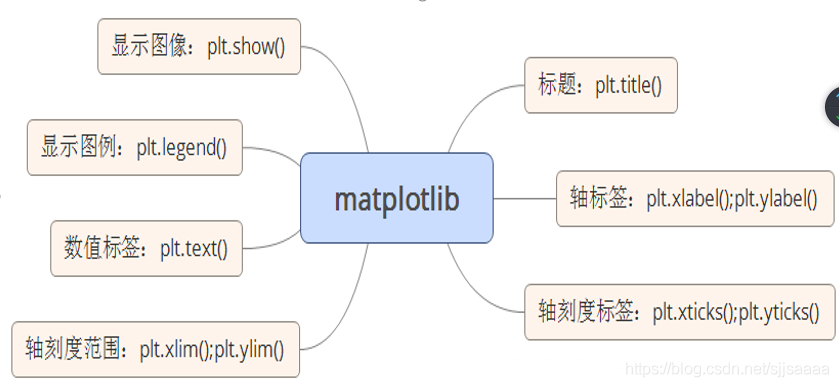• figure（）：创建一个新的绘图窗口。
• figtext（）：为figure添加文字
• axes（）：为当前figure添加一个坐标轴
• plot（）：绘图函数
• polar（）：绘制极坐标图
• axis（）：获取或设置轴属性的边界方法（坐标的取值范围）
• clf ： 清除当前figure窗口 cla ： 清除当前axes窗口
• close ： 关闭当前figure窗口
• subplot ： 一个图中包含多个axes
• text（）： 在轴上添加文字
• title（）： 设置当前axes标题
• xlabel/ylabel：设置当前X轴或Y轴的标签
• hist（）：绘制直方图
• hist2d（）：绘制二维在直方图
• hold ：设置当前图窗状态；off或者on
• legend（）：为当前axes放置标签
• pie（）：绘制饼状图
• scatter（）：做一个X和Y的散点图，其中X和Y是相同长度的序列对象
• stackplot（）：绘制一个堆叠面积图
• acorr（）：绘制X的自相关函数
• annotate（）：用箭头在指定的数据点创建一个注释或一段文本
• bar（）：绘制垂直条形图 barh（）：绘制横向条形图
• barbs（）：绘制一个倒钩的二维场

### 创建画布与创建子图

plt.figure 创建一个空白画布，可以指定画布大小，像素。

import matplotlib.pyplot as plt
import numpy as np
data=np.arange(10)
plt.plot(data)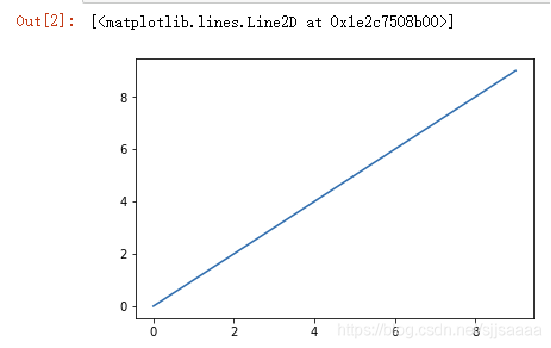import matplotlib.pyplot as plt
fig = plt.figure()
#不能使用空白的figure绘图，需要创建子图
ax3 = fig.add_subplot(2,2,3)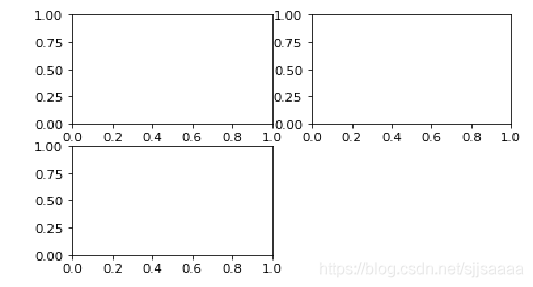fig = plt.figure()
ax1.plot([1.5,2,3.5,-1,1.6])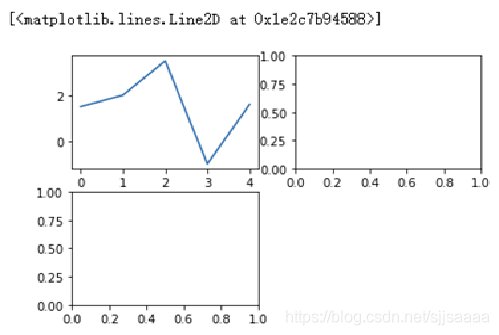fig , axes = plt.subplots(2,3)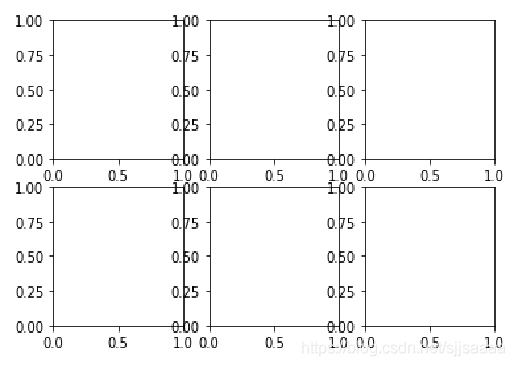fig,axes = plt.subplots(2,2,sharex = True,sharey = True)
for i in range(2):
for j in range(2):      axes[i,j].hist(np.random.randn(500),bins =50,color='k',alpha= 0.5)
plt.subplots_adjust(wspace=0,hspace=0)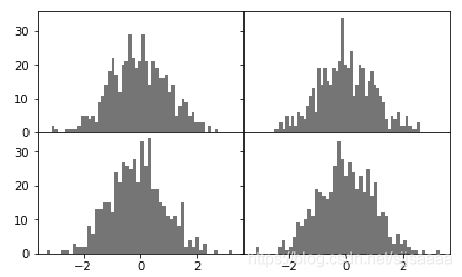### 添加画布内容

plt.title 在当前图形中添加标题，可以指定标题的名称、位置、颜色、字体大小等参数。
plt.xlabel 在当前图形中添加x轴名称，可以指定位置、颜色、字体大小等参数。
plt.ylabel 在当前图形中添加y轴名称，可以指定位置、颜色、字体大小等参数。
plt.xlim 定当前图形x轴的范围，只能确定一个数值区间，而无法使用字符串标识。
plt.ylim 指定当前图形y轴的范围，只能确定一个数值区间，而无法使用字符串标识。
plt.xticks 指定x轴刻度的数目与取值。
plt.yticks 指定y轴刻度的数目与取值。
plt.legend 指定当前图形的图例，可以指定图例的大小、位置、标签。

import numpy as np
import matplotlib.pyplot as plt
%matplotlib inline
data=np.arange(0,1,0.01)
plt.title('my lines example')
plt.xlabel('X')
plt.ylabel('Y')
plt.xlim(0,1)
plt.ylim(0,1)
plt.xticks([0,0.2,0.4,0.6,0.8,1])
plt.yticks([0,0.2,0.4,0.6,0.8,1])
plt.plot(data,data**2)
plt.plot(data,data**3)
plt.legend(['y=x^2','y=x^3'])
plt.show()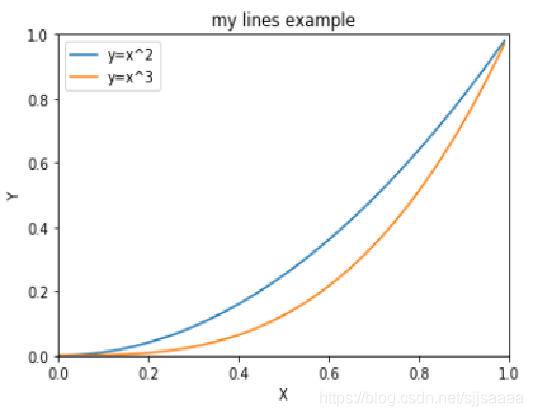import numpy as np
import matplotlib.pyplot as plt
%matplotlib inline
data=np.arange(0,np.pi*2,0.01)
fig1=plt.figure(figsize=(9,7),dpi=90)
#确定画布大小
#绘制第一幅子图
plt.title('lines example')
plt.xlabel('X')
plt.ylabel('Y')
plt.xlim(0,1)
plt.ylim(0,1)
plt.xticks([0,0.2,0.4,0.6,0.8,1])
plt.yticks([0,0.2,0.4,0.6,0.8,1])
plt.plot(data,data**2)
plt.plot(data,data**3)

#绘制第二幅子图
plt.title('sin-cos')
plt.xlabel('X')
plt.ylabel('Y')
plt.xlim(0,np.pi*2)
plt.ylim(-1,1)
plt.xticks([0,np.pi/2,np.pi,np.pi*3/2,np.pi*2])
plt.yticks([-1,-0.5,0,0.5,1])
plt.plot(data,np.sin(data))
plt.plot(data,np.cos(data))
plt.legend(['sin','cos'])
plt.show()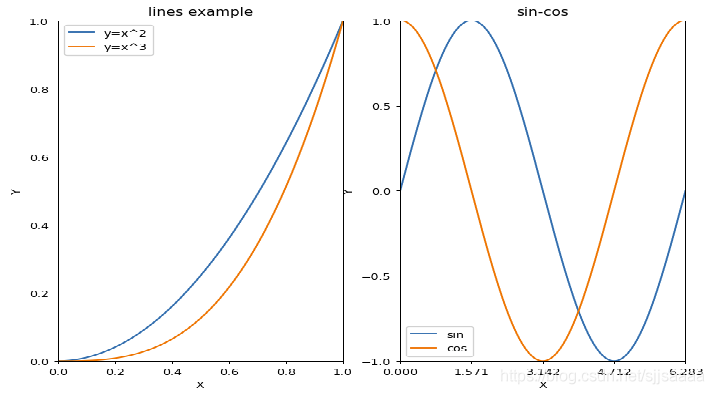Matplotlib 的 Legend 图例就是为了帮助我们展示每个数据对应的图像名称，更好的让读者认识到你的数据结构。关于plt.legend()的说明如下：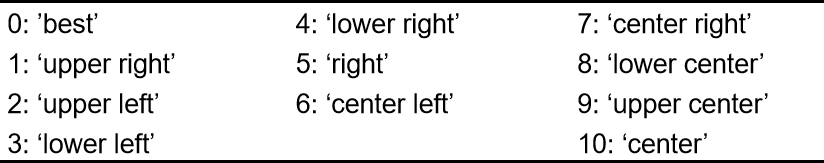plt.legend(loc = 'best',frameon = False)
#去掉图例边框,推荐使用
plt.legend(loc = 'best',edgecolor = 'blue')
#设置图例边框颜色
plt.legend(loc = 'best',facecolor = 'blue')
#设置图例背景颜色,若无边框,参数无效 

### 绘图的保存与显示

plt.savafig 保存绘制的图片，可以指定图片的分辨率、边缘的颜色等参数。
plt.show 在本机显示图形

fig.savefig(save_path, format='png', transparent=True, dpi=300, pad_inches = 0) 

figure.savefig选项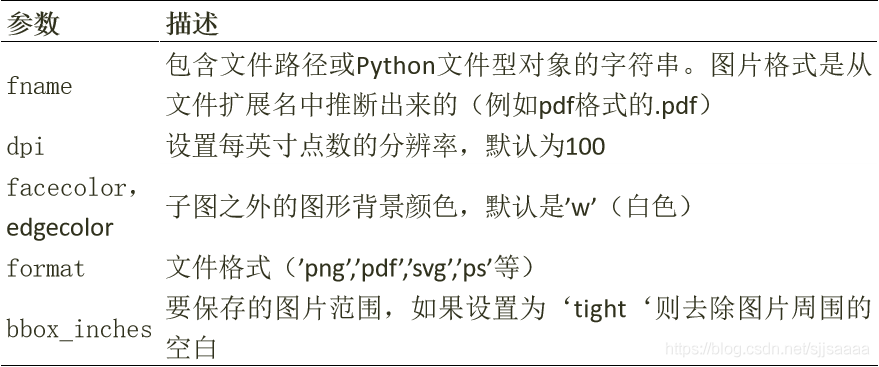## 3、rc参数设置

• pyplot使用rc配置文件来自定义图形的各种默认属性，被称为rc配置或rc参数。
• 在pyplot中几乎所有的默认属性都是可以控制的，例如视图窗口大小以及每英寸点数、线条宽度、颜色和样式、坐标轴、坐标和网格属性、文本、字体等。

 import matplotlib as plt
print(plt.rc_params()) 

import matplotlib as plt
print(plt.rc_params())

Axes：设置坐标轴边界、颜色、坐标

Figure：设置边界颜色、图形大小和子区；
Font：设置字号、字体和样式；
Grid：设置网格颜色和线型；
Legend：设置图例和其中的文本显示；
Lines：设置线条颜色、宽度、线型等；
Savefig：对保存图像进行单独设置；
Xtick和ytick：X、Y轴的主刻度和次刻度设置颜色、大小、方向和标签大小。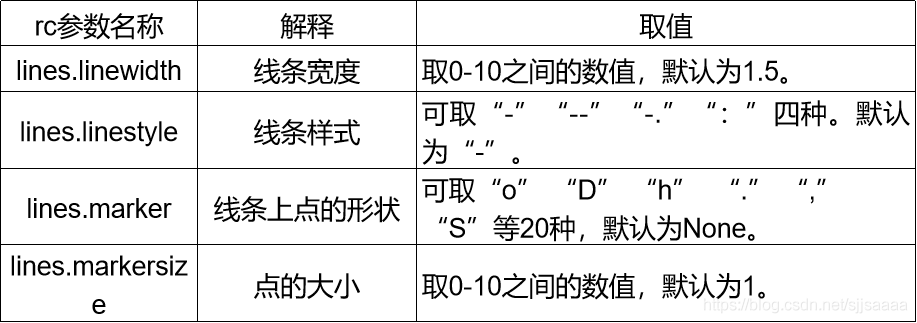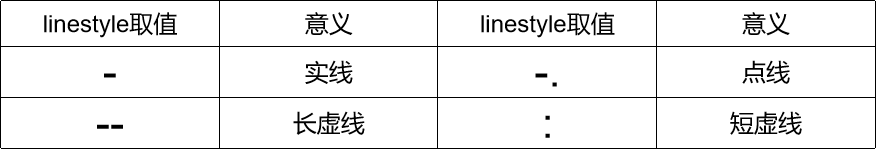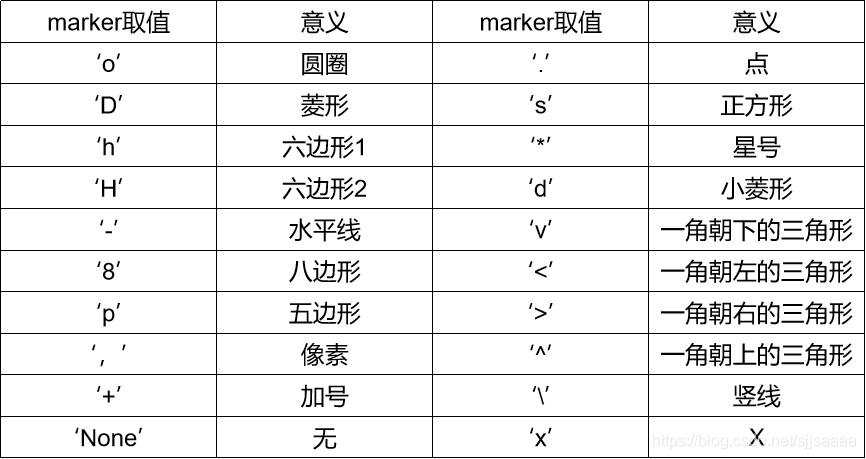import numpy as np
import matplotlib.pyplot as plt
fig,ax = plt.subplots()
#配置中文显示
plt.rcParams['font.family'] = ['SimHei']
plt.rcParams['axes.unicode_minus'] = False
def f(t):
return np.cos(2*np.pi*t)
x1 = np.arange(0.0,4.0,0.5)
x2 = np.arange(0.0,4.0,0.01)
plt.figure(1)
plt.subplot(2,2,1)
plt.plot(x1,f(x1),'bo',x2,f(x2),'k')
plt.title('子图1')
plt.subplot(2,2,2)
plt.plot(np.cos(2*np.pi*x2),'r--')
plt.title('子图2')
plt.show()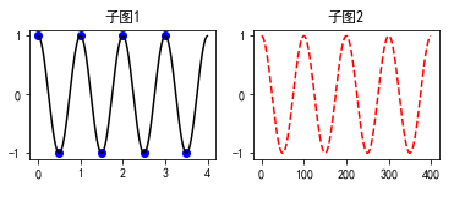import numpy as np
import matplotlib.pyplot as plt
fig,ax=plt.subplots()
#配置中文显示
plt.rcParams['font.family']=['SimHei']#用来显示中文标签
plt.rcParams['axes.unicode_minus']=False  #用来正常显示负号
def f(t):
return np.cos(2*np.pi*t)

x1=np.arange(0.0,5.0,0.5)
x2=np.arange(0.0,5.0,0.01)
plt.figure(1)
plt.subplot(2,2,1)
plt.plot(x1,f(x1),'bo',x2,f(x2),'k')
plt.subplot(2,2,2)
plt.plot(np.cos(2*np.pi*x2),'r--')
plt.show()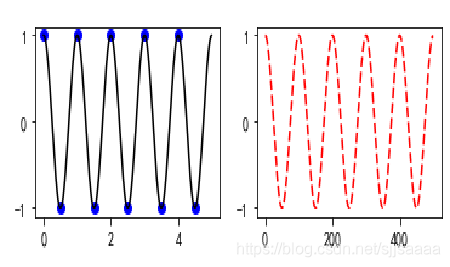1. 调用函数fill_between() 实现曲线下面部分的填充：
import numpy as np
import matplotlib.pyplot as plt
x=np.linspace(0,1,500)
y=np.sin(3*np.pi*x)*np.exp(-4*x)
fig,ax=plt.subplots()
plt.plot(x,y)
plt.fill_between(x, 0, y, facecolor='green', alpha=0.3)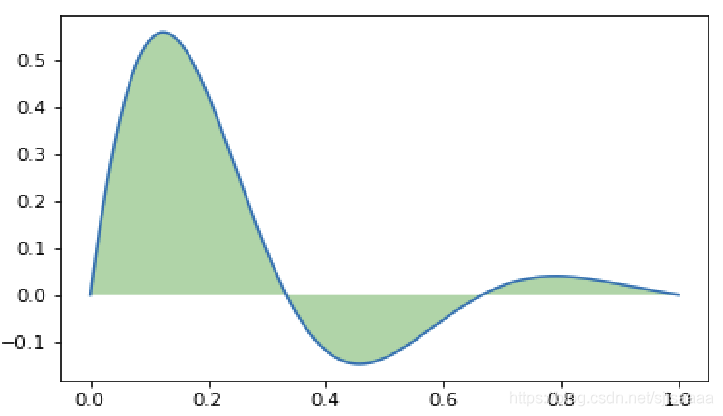• x：第一个参数表示覆盖的区域， x，表示整个x都覆盖
• 0：表示覆盖的下限
• y：表示覆盖的上限是y这个曲线
• facecolor：覆盖区域的颜色
• alpha：覆盖区域的透明度[0,1],其值越大，表示越不透明
1. 部分区域的填充：
plt.fill_between(x[15:300], 0, 0.4, facecolor='green', alpha=0.3)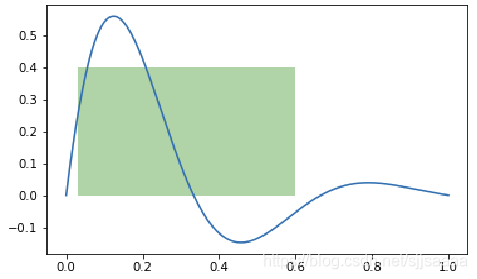1. 两条曲线之间的区域填充：
import numpy as np
import matplotlib.pyplot as plt
x=np.linspace(0,1,500)
y1=np.sin(3*np.pi*x)*np.exp(-4*x)
y2 = y1 + 0.2
plt.plot(x, y1,'b')
plt.plot(x, y2, 'r')
plt.fill_between(x, y1, y2, facecolor='green', alpha=0.3)
plt.show()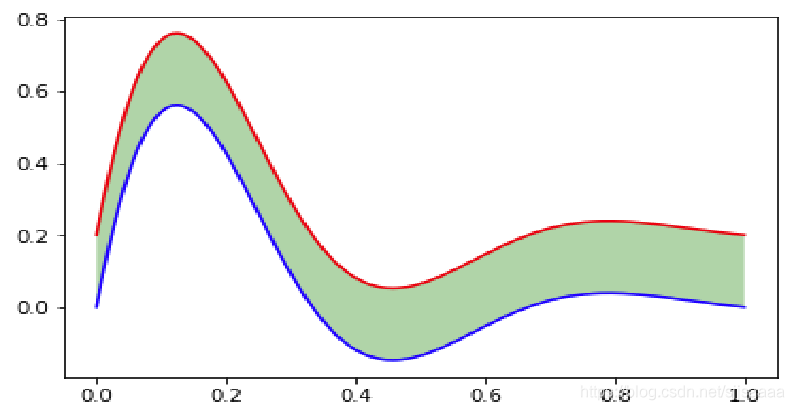4. 利用fill进行绘图的填充：

import numpy as np
import matplotlib.pyplot as plt
x=np.linspace(0,1,500)
y=np.sin(3*np.pi*x)*np.exp(-4*x)
fig,ax=plt.subplots()
ax.fill(x,y)
plt.show()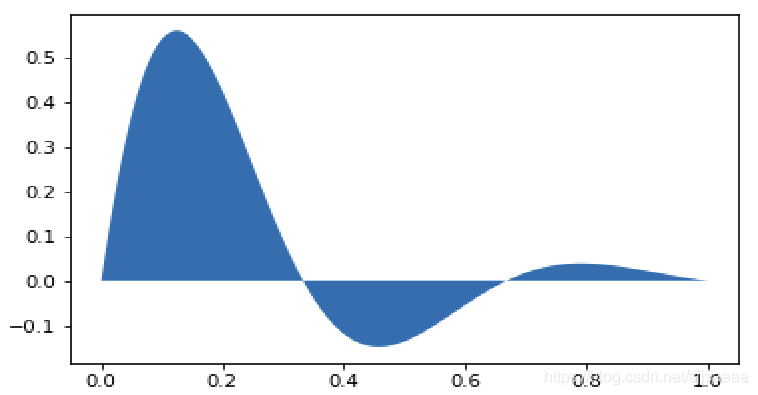import numpy as np
import matplotlib.pyplot as plt
plt.rcParams['font.family'] = ['SimHei']
data = [25,30,32,34,34,23]
label = ['青海','兰州','北京','上海','广州','拉萨']
plt.xticks(range(len( data)),label)
plt.xlabel('城市')
plt.ylabel('温度')
plt.title('六城市8月份日均最高气温')
plt.bar(range(len( data)),data)
for x,y in zip(range(len(data)),data):
plt.text(x,y,y,ha = 'center',va = 'bottom')
plt.show()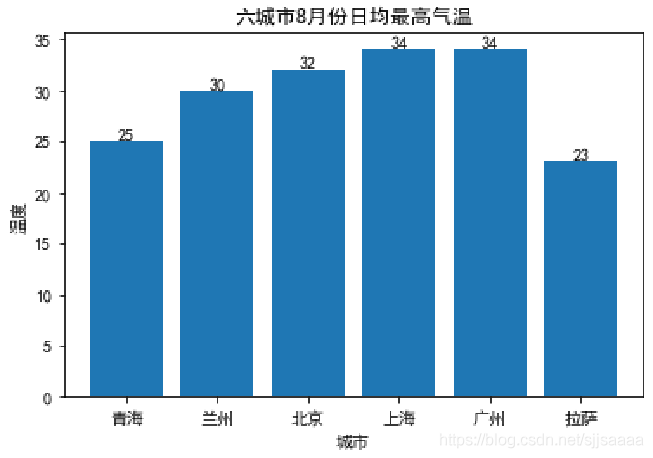## 4、pyplot中的常用绘图

### 折线图

plot函数：matplotlib.pyplot.plot(*args, **kwargs)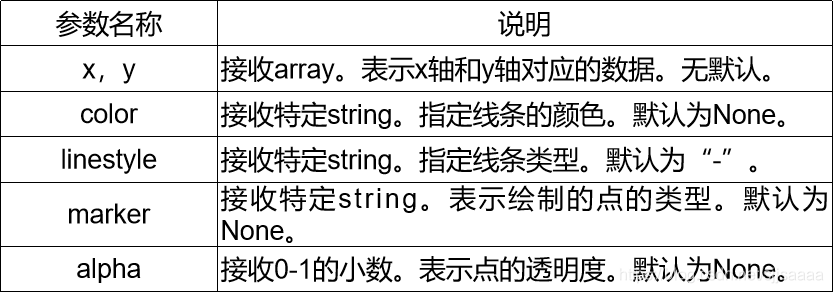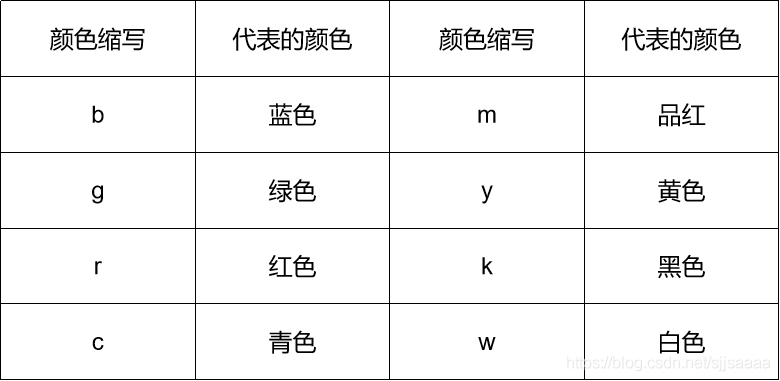带点的折线图
import numpy as np
x1 = np.arange(0, 30)
plt.plot(x1,x1*2, 'b')
plt.plot(x1,x1*2, 'bo')
plt.show()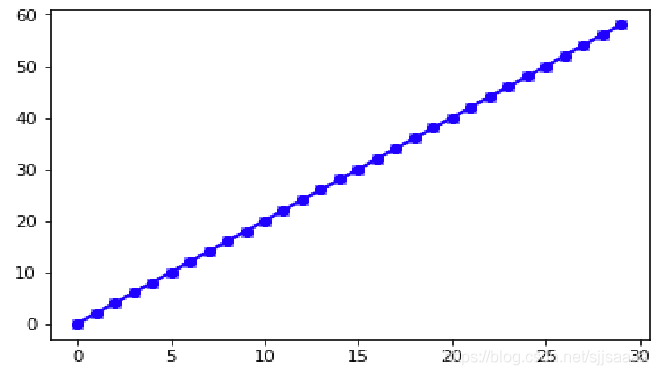### 散点图

scatter函数：
matplotlib.pyplot.scatter(x, y, s=None, c=None, marker=None, alpha=None, **kwargs)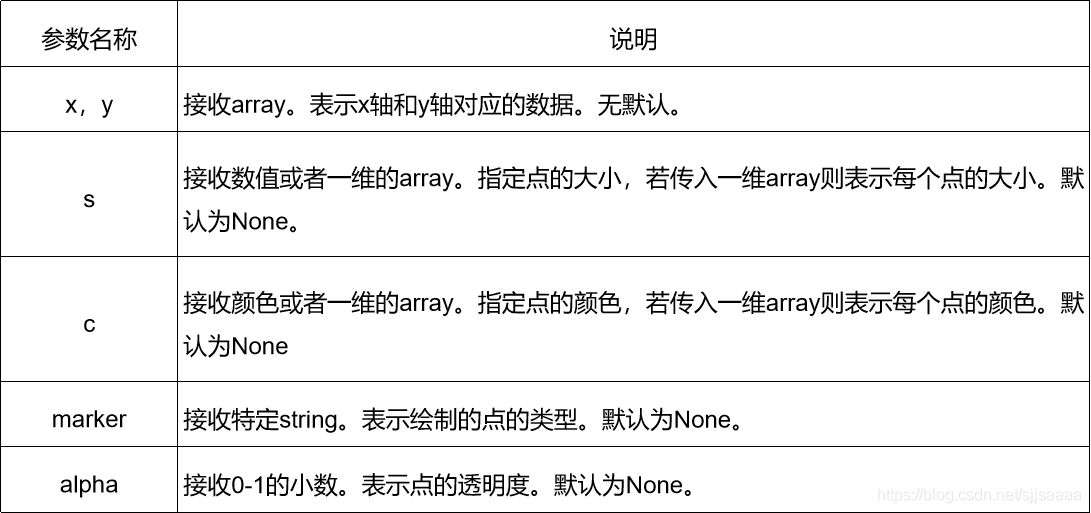import numpy as np
import matplotlib.pyplot as plt
fig,ax=plt.subplots()
plt.rcParams['font.family']=['SimHei']#用来显示中文标签
plt.rcParams['axes.unicode_minus']=False  #用来正常显示负号
x1=np.arange(1,30)
y1=np.sin(x1)

ax1=plt.subplot(1,1,1)
plt.title('散点图')
plt.xlabel('X')
plt.ylabel('Y')
lvalue=x1
ax1.scatter(x1,y1,c='r',s=100,linewidths=lvalue,marker='o')
plt.legend('x1')
plt.show()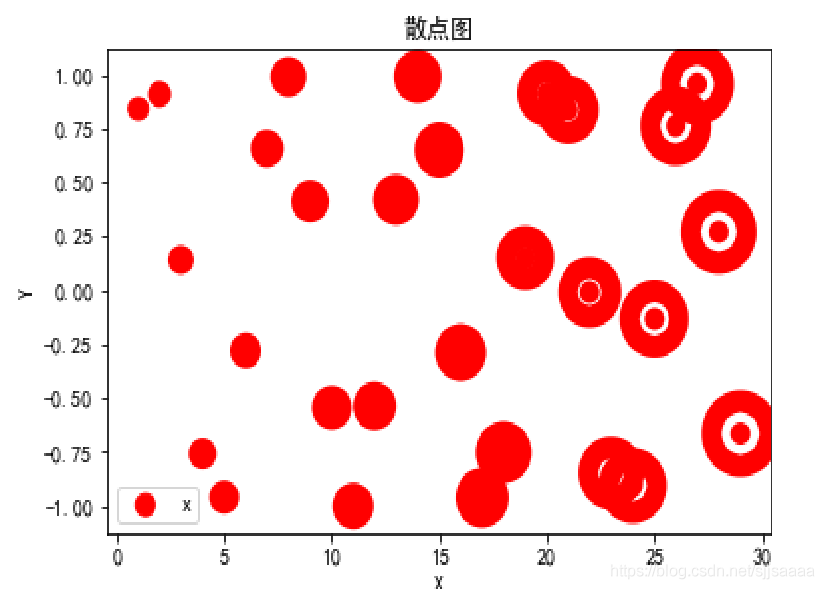import numpy as np
import matplotlib.pyplot as plt
fig,ax=plt.subplots()
plt.rcParams['font.family']=['SimHei']#用来显示中文标签
plt.rcParams['axes.unicode_minus']=False  #用来正常显示负号
for color in ['red','green','blue']:
n=500
x,y=np.random.randn(2,n)    ax.scatter(x,y,c=color,label=color,alpha=0.3,edgecolors='none')
ax.legend()
ax.grid(True)

plt.show()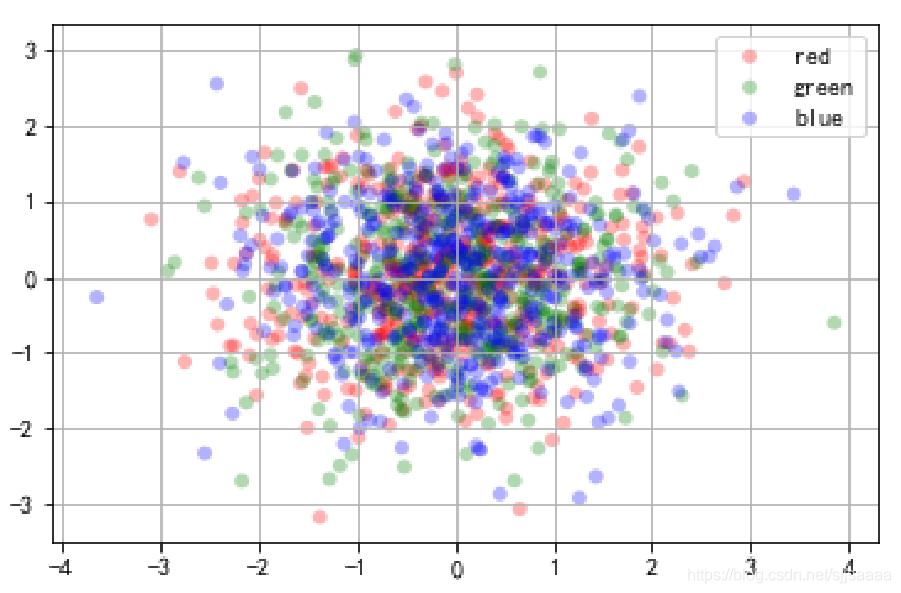### 直方图

bar函数：
matplotlib.pyplot.bar（left，height，width = 0.8，bottom = None，hold = None，data = None，** kwargs ）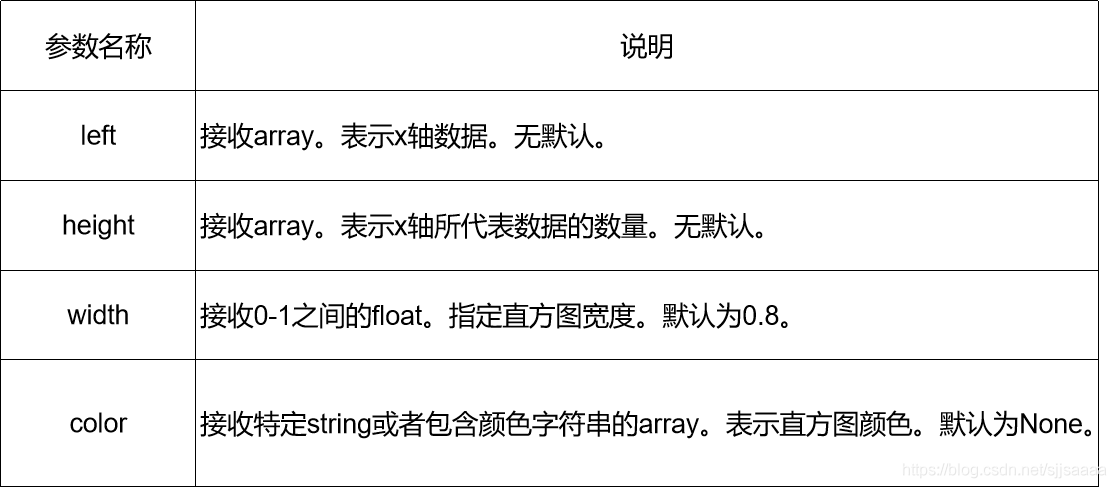fig,axes=plt.subplots(2,1)
data=pd.Series(np.random.randn(16),index=list('abcdefghijklmnop'))
data.plot.bar(ax=axes,color=‘k’,alpha=0.7) #垂直柱状图
data.plot.barh(ax=axes,color=‘k’,alpha=0.7) #alpha设置透明度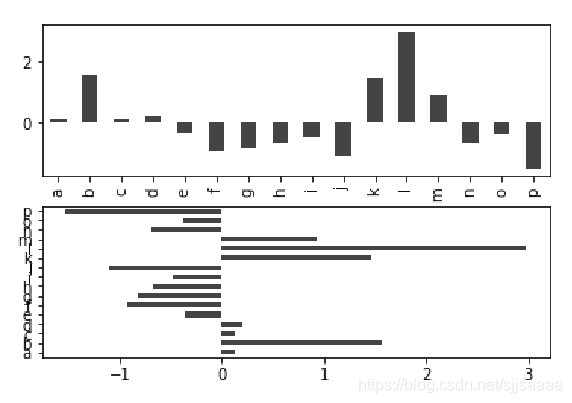import numpy as np
import matplotlib.pyplot as plt
fig,ax=plt.subplots()
plt.rcParams['font.family']=['SimHei']#用来显示中文标签
plt.rcParams['axes.unicode_minus']=False  #用来正常显示负号
plt.figure(figsize=(7,5))
x=np.arange(1,6)
Y1=np.random.uniform(1.5,1.0,5)
Y2=np.random.uniform(1.5,1.0,5)
plt.bar(x,Y1,width=0.35,facecolor='lightskyblue',edgecolor='white')
plt.bar(x+0.35,Y2,width=0.35,facecolor='yellowgreen',edgecolor='white')
plt.show()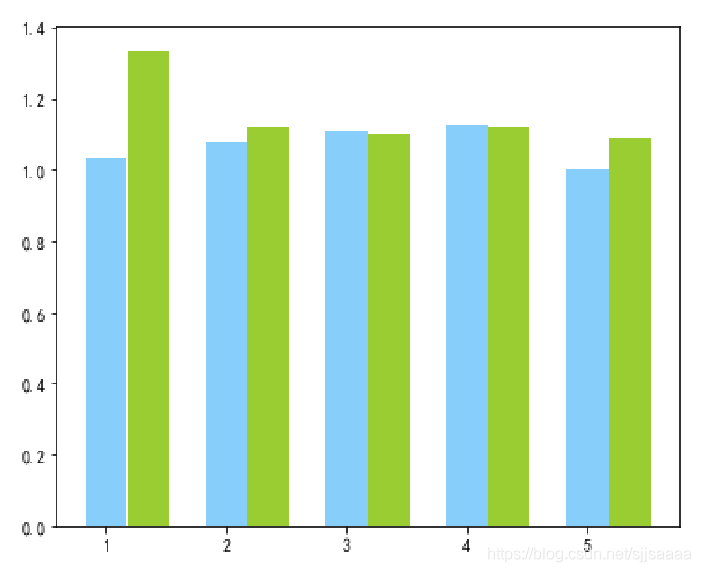### 饼图

pie函数
matplotlib.pyplot.pie(x, explode=None, labels=None, colors=None, autopct=None, pctdistance=0.6, shadow=False, labeldistance=1.1, startangle=None, radius=None, … )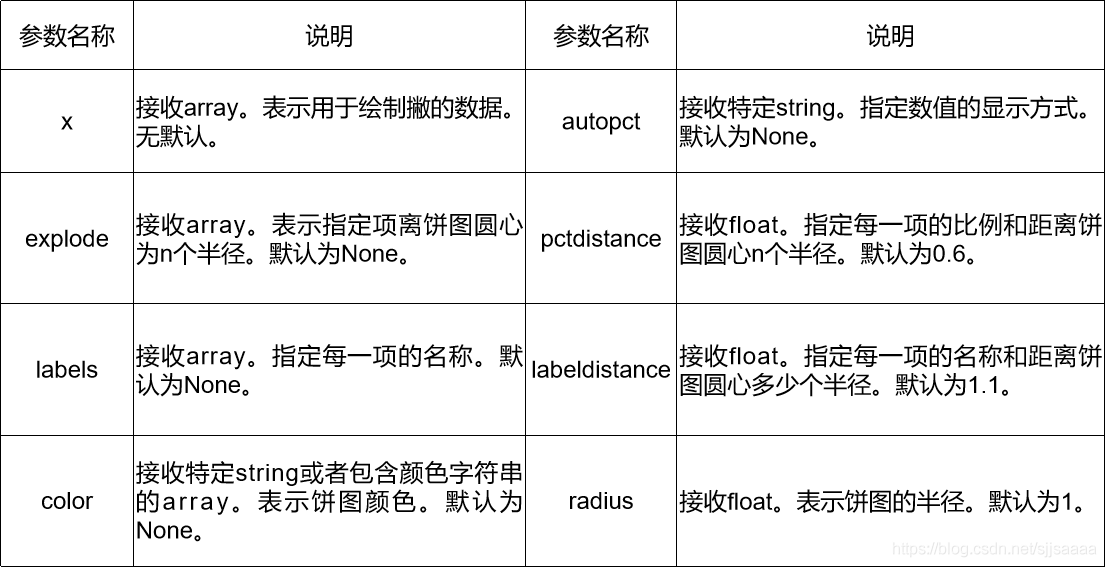plt.figure(figsize=(6,6))
ax=plt.axes([0.1,0.1,0.8,0.8])#建立轴的大小
labels='Springs','Summer','Autumn','Winter'
x=[15,30,45,10]
explode=(0.05,0.05,0.05,0.05)#这个是控制分离的距离的，默认的饼图不分离。
plt.pie(x,labels=labels,explode=explode,startangle=60,autopct='%1.1f%%')#qutopct在图中显示比例值，注意值的格式。
plt.title('Rany days by season')
plt.show()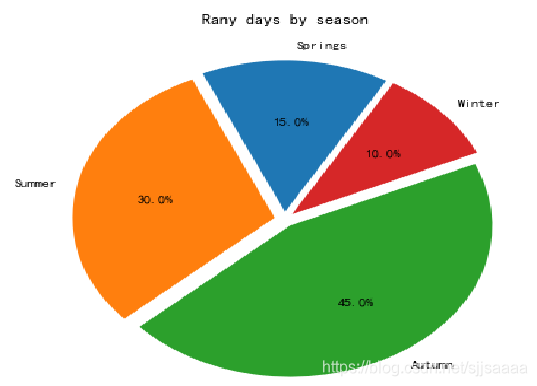### 箱线图

boxplot函数
matplotlib.pyplot.boxplot(x, notch=None, sym=None, vert=None, whis=None, positions=None, widths=None, patch_artist=None,meanline=None, labels=None, … )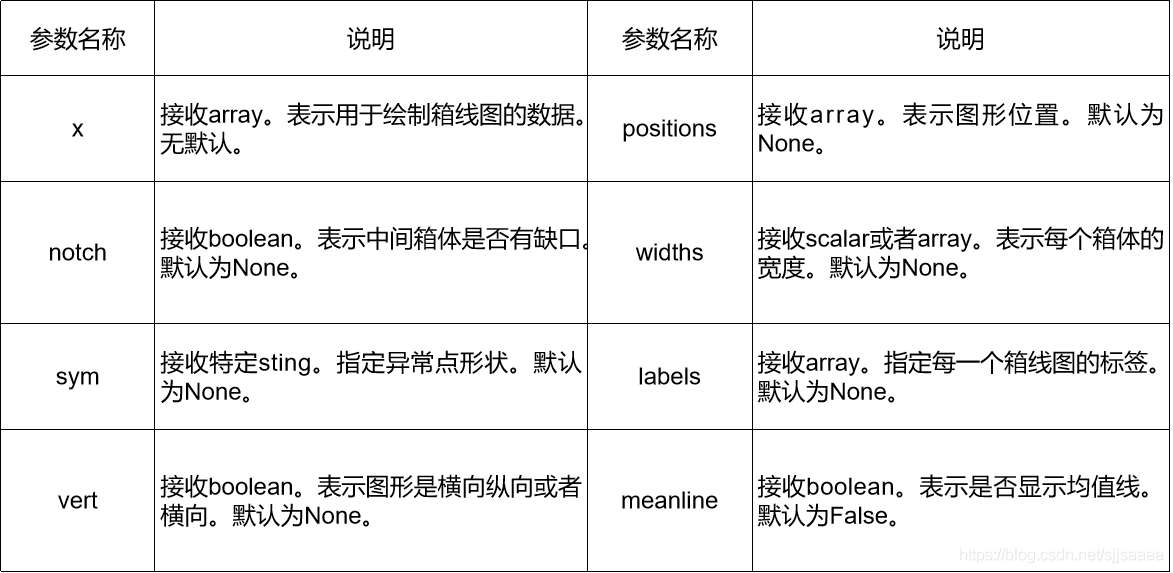import numpy as np
import matplotlib.pyplot as plt
import pandas as pd
np.random.seed(2)  #设置随机种子
df = pd.DataFrame(np.random.rand(5,4),
columns=['A', 'B', 'C', 'D'])#先生成0-1之间的5*4维度数据，再装入4列DataFrame中
df.boxplot() #也可用plot.box()
plt.show()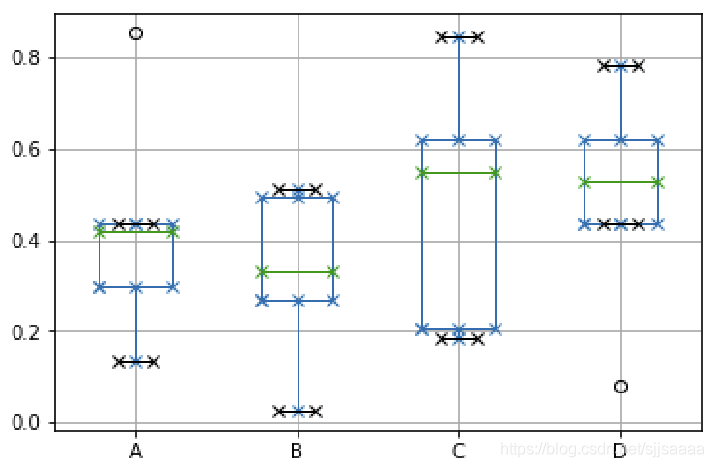### 概率图

import numpy as np
import matplotlib.pyplot as plt
fig,ax=plt.subplots()
plt.rcParams['font.family']=['SimHei']#用来显示中文标签
plt.rcParams['axes.unicode_minus']=False  #用来正常显示负号
np.random.seed(1587554)
mu=100
sigma=15
x=mu+sigma*np.random.randn(437)
num_bins=50
n,bins,patches=ax.hist(x,num_bins,normed=1)
y=plt.mlab.normpdf(bins,mu,sigma)
ax.plot(bins,y,'--')
fig.tight_layout()
plt.show()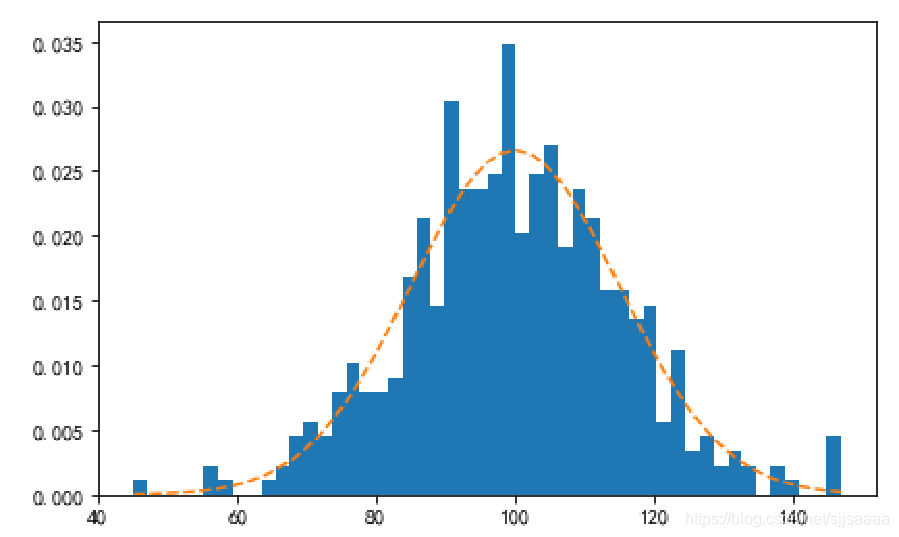## 5、利用Matplotlib绘制词云

（1）WordCloud
（2）jieba
conda install -c conda-forge jieba

（1）首先使用pandas读取数据并将需要分析的数据转化为列表；
（2）对获得的列表数据利用分词工具jieba进行遍历分词；
（3）使用WordCloud设置词云图片的属性、掩码和停用词，并 生成词云图像。

def get_wordClound(mylist):
word_list = [" ".join(jieba.cut(sentence))for sentence in mylist]
new_text = ' '.join(word_list)
get_wordClound(wordList)